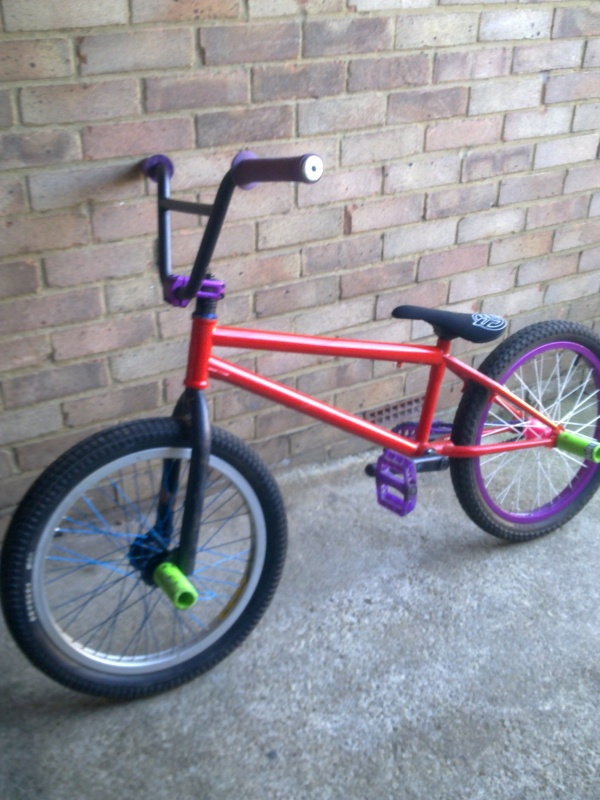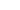• + 1DirtBikeer (Mar 23, 2010 at 13:47)
what i meant was ur new version
• + 1umar123 (Mar 23, 2010 at 13:53)
i havnt changed anything frm this bike, cba lol
• + 1DirtBikeer (Mar 24, 2010 at 13:29)
i thought u had i must be loasing my mind.
• + 1umar123 (Mar 25, 2010 at 1:02)
yer lol
• + 1DirtBikeer (Mar 23, 2010 at 13:30)
• + 1umar123 (Mar 23, 2010 at 13:42)
this is ma bike• + 1schilvers (Feb 16, 2010 at 7:57)
frame 50 posted with bb?
• + 1umar123 (Feb 16, 2010 at 11:57)
no mate. its worth alot mree
• + 1schilvers (Feb 16, 2010 at 16:37)
80?
• + 1umar123 (Feb 17, 2010 at 3:45)
no lol
• + 1eldavo14 (Feb 14, 2010 at 10:30)
sweet ride man
• + 1umar123 (Feb 14, 2010 at 11:31)
thanks man
• + 1bikerdude1993 (Feb 16, 2010 at 1:32)
what hub is it?
• + 1umar123 (Mar 23, 2010 at 13:42)
Ody V3
• + 1kian95 (Feb 14, 2010 at 10:22)
omg thats soooo nice
• + 1umar123 (Feb 14, 2010 at 11:31)
thanks
• + 1umar123 (Feb 14, 2010 at 11:32)
Not even done yet ha
• + 1kian95 (Feb 14, 2010 at 12:16)
why what else you doin?
• + 1umar123 (Feb 14, 2010 at 14:01)
New front wheel, new forks, new headet, new bars
• + 1kian95 (Feb 14, 2010 at 14:38)
would you sell them bars?
• + 1umar123 (Feb 15, 2010 at 3:45)
yer
• + 1kian95 (Feb 15, 2010 at 6:38)
how much
• + 1umar123 (Feb 15, 2010 at 9:01)
£20
• + 1kian95 (Feb 15, 2010 at 9:21)
posted?
• + 1kian95 (Feb 15, 2010 at 9:22)
and with grips?
• + 1umar123 (Feb 15, 2010 at 9:28)
na mate.